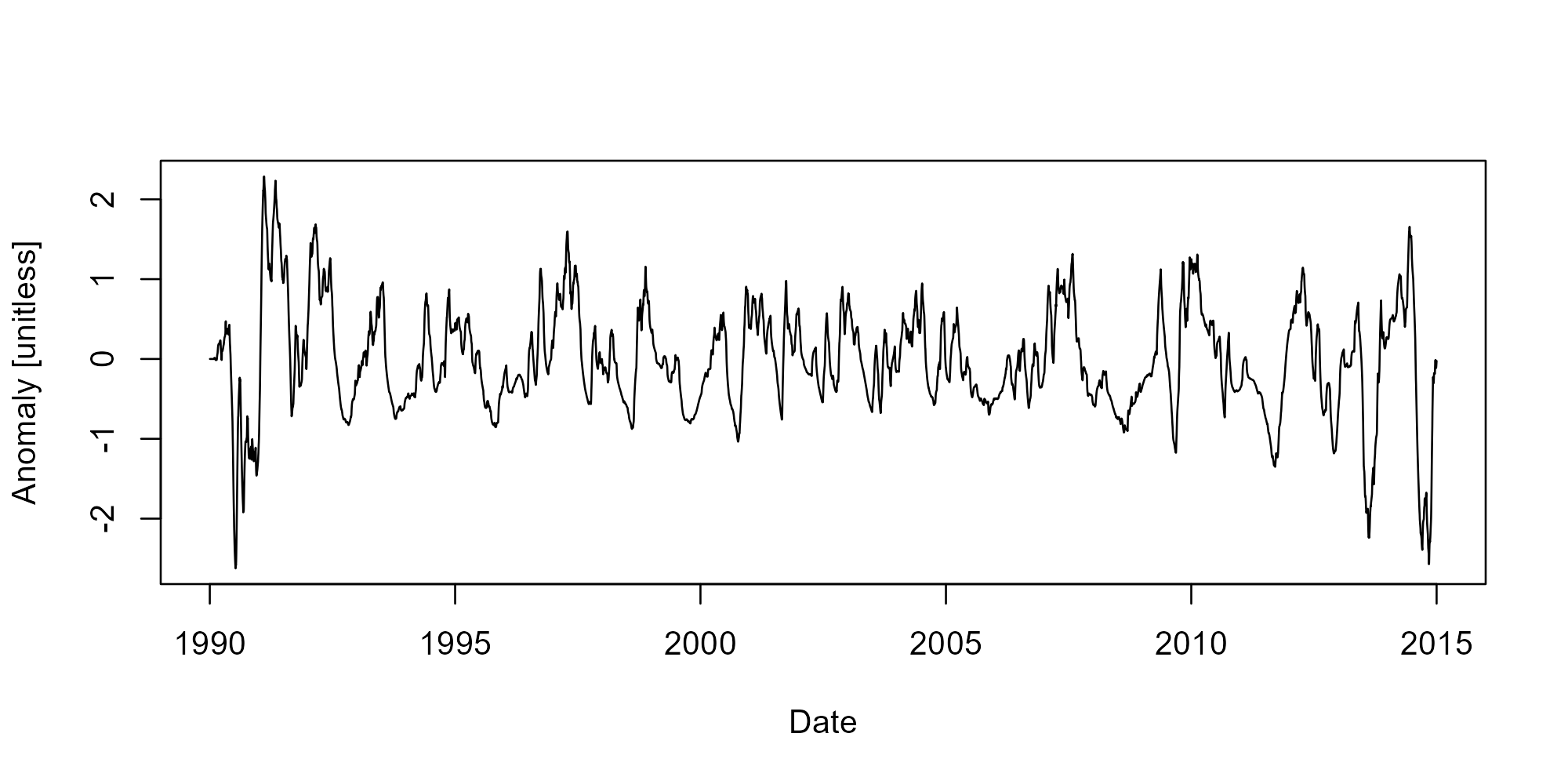adc provides functions to calculate discharge-based metrics that are useful in water quality concentration and flux regression equations.

## Installation

``install.packages('adc')``

The development version is available on https://txwri.r-universe.dev/adc and can be installed with:

``install.packages('adc', repos = c(txwri = 'https://txwri.r-universe.dev'))``

## Example

Flow anomalies represent how different the current discharge period is (current day, current week, current month, etc.) from previous periods (previous week, previous month, period of record, etc.).

``````library(adc)
## example code is lavaca and includes dates and mean daily flow

data(lavaca)

x <- fa(lavaca\$Flow,
dates = lavaca\$Date,
T_1 = "1 month",
T_2 = "1 year",
clean_up = TRUE,
transform = "log10")

plot(lavaca\$Date, x, type = "l", xlab = "Date", ylab = "Anomaly [unitless]")``````The packages also includes functions to calculate an exponentially weighted discounted flow, base-flow, and rate of change for mean daily streamflow. Functions generally work well using the `dplyr::mutate()` function to facilitate tidy workflows.